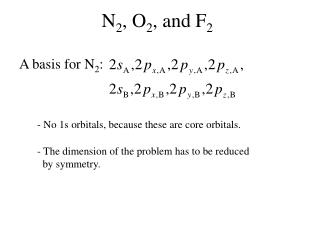DownloadDownload PresentationN 2 , O 2 , and F 2

# N 2 , O 2 , and F 2

Download Presentation## N 2 , O 2 , and F 2

- - - - - - - - - - - - - - - - - - - - - - - - - - - E N D - - - - - - - - - - - - - - - - - - - - - - - - - - -
##### Presentation Transcript

1. A basis for N2: N2, O2, and F2 - No 1s orbitals, because these are core orbitals. - The dimension of the problem has to be reduced by symmetry.

2. N2, O2, and F2 (continued) Intermezzo: The 2p orbitals.

3. N2, O2, and F2 (continued) Intermezzo: The 2p orbitals.

4. N2, O2, and F2 (continued) A B Symmetry elements:

5. N2, O2, and F2 (continued) A B Make plus and minus combination from orbitals that transform into each other.

6. N2, O2, and F2 (continued) combination group I II III IV V VI II I

7. N2, O2, and F2 (continued) group I: group II: group III: group IV: group V: group VI: - There is no interaction between orbitals of different groups. - The eight-dimensional problem is reduced to six smaller problems. - The one-dimensional problems directly give solutions of the Fock-equation. - Groups III and V, and groups IV and VI give degenerate solutions.

8. N2, O2, and F2 (continued) Bond-order = (# bonding electrons - # antibonding electrons)/2 = (8 - 2) / 2 = 3 N N2 N

9. N2, O2, and F2 (continued) MO of N2:

10. N2, O2, and F2 (continued) MO of N2:

11. N2, O2, and F2 (continued) MO of N2:

12. N2, O2, and F2 (continued) MO of N2:

13. N2, O2, and F2 (continued) MO of N2:

14. N2, O2, and F2 (continued) MO of N2:

15. N2, O2, and F2 (continued) O O2 O Bond-order = (# bonding electrons - # antibonding electrons)/2 = (8 - 4) / 2 = 2

16. N2, O2, and F2 (continued) Hund’s second rule: The Slater-determinant has the lowest energy if electrons are distributed as much as possible over degenerated orbitals. Hund’s third rule: The Slater-determinant has the lowest energy if electrons are placed in degenerated orbitals with the same spin.

17. N2, O2, and F2 (continued) F F2 F Bond-order = (# bonding electrons - # antibonding electrons)/2 = (8 - 6) / 2 = 1